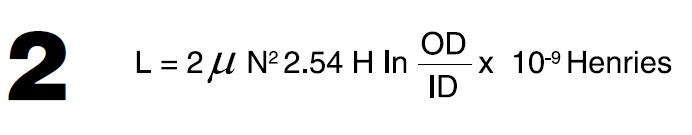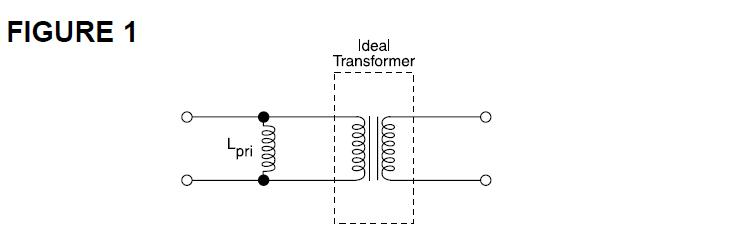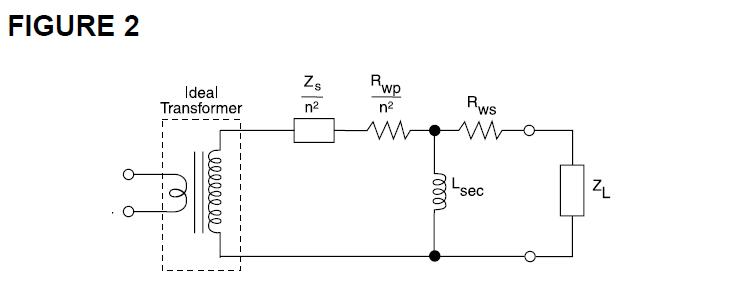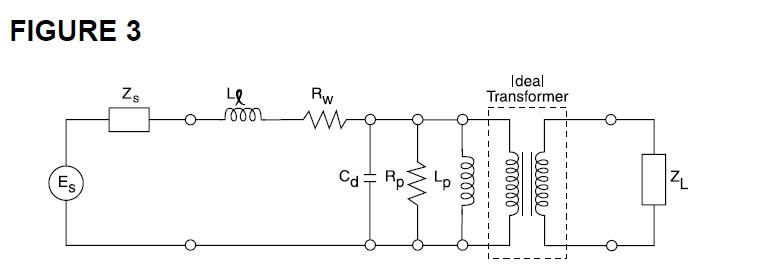Design Considerations of Toroid Transformers and other magnetic devices - Manufacturing in China Blog Google+

# Manufacturing in China Blog

Here you can leave comments and start discussions about manufacturing parts of complete products in China.

# Design Considerations of Toroid Transformers and other magnetic devices

Posted by P&A InternationalP&A International
P&A International is China based company formed by partners with engineering background in 2008. Our main focu...
User is currently offline
on Tuesday, 06 November 2012 in General Talk

Ferrite toroid transformers provide an often convenient and very effective shape for many wide band, pulse and power transformers and inductors. The continuous magnetic path yields the highest effective permeability and lowest flux leakage of any shape. Basic considerations: The inductance may be calculated from(the units are in CGS system which is used throughout this catalog). Here le and Ae are the effective magnetic path length and cross sectional area of the core, . is the effective permeability of the material, and N is the number of turns. This formula may be used for any shape under all conditions provided the correct value of . is used and stray reactances are given proper consideration. In a toroidal core, this may be expressed as:where OD, ID and H are the dimensions in inches. For low level conditions at comparatively low frequencies the formula may be simplified by using the Inductance Index, AL, listed in this catalog. Then;The other value most frequently needed is peak flux density, which may be calculated fromHere E is the RMS voltage, 4.44 is a constant depending on the wave shape (use 4 when E is symmetrical square wave and 1 where E is a unipolar pulse), and f is the frequency in hertz.

This section considers those applications where nonlinearity and losses due to hysteresis are negligible. Generally this means flux densities below a few hundred Gauss. The first material choice is the one having both the highest permeability and lowest loss factor, tan ./., at the operating frequency. Considering the space available, select a core from the table and, using its inductance index, AL, calculate the number of turns required to give the desired inductance. Now select the largest practical wire size that will fit on the core. This is somewhat difficult for a toroid, but generally the total wire cross section in the winding can be 30-60% of the window opening. If there are Q or loss requirements calculate the resistance of the winding, taking into consideration the skin effect if the frequency is high, and add it to the equivalent series resistance contributed by the core losses. Equation 5 shows the relationship between loss factor, Q and resistance.If the calculated Q is inadequate you must reduce the total series resistance by selecting a larger core that will allow fewer turns of larger wire, select a less lossy material, or use Litz wire at high frequencies to minimize the skin effect.If losses are critical it is important to remember that hysteresis losses have been assumed to be negligible. Above a few 10’s of Gauss these losses are measurable and increase as approximately the 2.5 power of flux density. Also, remember that ferrites like other magnetic materials show variation in inductance from part to part, with temperature and with magnetizing force. Unlike powdered metals which have air gaps between the particles a ferrite toroid is a continuous magnetic material with variability effects undiluted by air gaps. This means that tight tolerances such as required for wave filters are not attainable in a toroid, but will generally require a gapped structure such as an E core, pot core, or slug.

POWER INDUCTORS:
In this section we consider inductors where the design is limited by saturation or heating due to core or winding losses. Although there is no systematic connection between permeability and losses, below about 1 MHz relatively high permeability manganese-zinc ferrites have the most desirable combination of high saturation flux density and low hysteresis losses. The first step is to select one of those materials having the desired properties (usually B material) and select a core based on space limitations. Then select a suitable operating flux density. As a general rule, at room temperature materials may be operated to the knee of the BH loop when the frequency is 20 kHz or less. At higher frequencies hysteresis losses produce enough heat to require that the flux density be decreased. As a first approximation, the product of flux density and frequency can be held constant above 20 kHz. Knowing the voltage, frequency, flux density and area of the chosen core the minimum number of turns may be calculated from equation 4. The inductance can then be estimated from equation 3 or calculated more exactly from equations 1 or 2 by using the appropriate value of permeability under these operating conditions. If this inductance is less than the desired value, the number of turns can be adjusted upward provided there is sufficient space for the winding. If the inductance is too great it will be necessary to choose a larger core whose cross sectional area is greater but whose ratio of Ae/le is less, or a material with lower permeability. For inductors operating above 1 MHz the material choice becomes more difficult since other requirements such as return loss may be more important. The material choice and design procedure will depend on which factors predominate in your particular design. Inductors having dc current superimposed on the ac excitation must be given special treatment. The magnetizing force may be calculated using equation 6:With this information it is possible to estimate from the BH curves how significant will be the effect of the dc current. Generally dc magnetizing forces less the coercive force will have only a small effect on permeability, moderate values will depress the permeability, and magnetizing forces approaching the knee of the BH loop will considerably reduce the permeability and severely limit the peak flux density available for ac excitation. In these cases, unless a higher inductance can be used it will be necessary to go to a core with a considerably longer magnetic path length or to provide an air gap such as by slotting the core.

In many power applications thermal considerations control the design. One rule of thumb that may be useful for first approximations is that core losses of 100 to 600 mW/cm3 produce an approximate 40° C temperature rise. The exact value depends on inductor geometry and thermodynamic considerations beyond the scope of this guide. You must also consider the power dissipated in the winding and its contribution to inductor heating. Heat sinking or coolants may be used to remove this heat, but the thermal conductivity of ferrite is relatively low, so the interior core temperature will be higher. Should a large temperature gradient develop, the core may crack from thermal stresses. Also, where considerable temperature excursions occur due either to self heating or ambient temperatures, the effect of these changes must also be considered with respect to changes in saturation flux density and inductance.

LOW LEVEL TRANSFORMERS:

The design procedure here is essentially the same as for low level inductors except, of course, that the winding space must be shared between the primary and secondary windings. Usually half the space is allotted to each. In selecting the inductance required it is easiest to envision the equivalent circuit as an ideal transformer (figure 1) with a primary self inductance shunting the transformer primary. When the impedance represented by this inductance is high compared to the primary and transformed secondary impedances it may be neglected and an ideal transformer results. Ordinarily this impedance is selected to be between 3 and 10 times the source impedance. At very high frequencies losses or winding capacitance and leakage inductance may predominate.These situations are considered in the later paragraphs.

CURRENT TRANSFORMERS:

This special class of low level (and sometimes power) transformers includes ground fault interrupters (GFI) sensors. In this case it is simpler to design around the secondary. Because there are often few primary turns (usually one) and many secondary turns, the transformed source impedance (Zs) and primary winding resistance (Rwp) can not always be neglected. As shown in figure 2, these impedances are increased by 1/n2 (where n is the primary to secondary turns ratio) .As n is decreased to raise the secondary voltage all four internal impedances shown in figure 2 increase. This limits the available load voltage, so a compromise must be made for optimum performance. Since the core losses of high permeability ferrites are small at audio frequencies, they may often be neglected. For this reason, ferrite toroids are usually selected for grounded neutral transformers in GFl’s-particularly when frequencies above 60 Hz are used for this test. Special manufacturing and test techniques can be used to enhance the properties of ferrite toroids for GFI differential fault transformers, as well.

Here we are considering the same kinds of situations we covered under power inductors, that is, those cases where the design is limited by saturation flux density or self heating due to core and winding losses. At low frequencies, say below 1 MHz, the design procedure is the same as that for power inductors except, of course, that winding space must be allowed for both windings. Ordinarily allot half each to the primary and secondary, or with a push-pull primary, slightly less than one third to each primary half. In most cases the voltage and frequency are known (use the lowest operating frequency for design purposes). Select a material and flux density in the same manner as for power inductors. Then using equation 4, calculate the product of Ae and N required. It is then a simple matter to go down the list of suitable core sizes substituting for Ae, calculating the minimum number of turns required and checking the fit of the winding in that core. Calculating the primary inductance from equations 1, 2, or 3, you will ordinarily find that the inductance will be large enough that the magnetizing current may be neglected under full load. (This is the current drawn by the primary inductance which shunts the ideal transformer.) The rest of the transformer design is fairly straight-forward and is covered in other publications. Most devices of this type are limited by either saturation or heat dissipation, temperature rise and efficiency. Often winding losses are greater than core losses below 50 kHz. In some cases other considerations such as regulation may take precedence, but the considerations described above must still be met. At higher frequencies in the MHz range other factors such as eddy currents influence the design. For this reason higher resistivity nickel zinc ferrites are ordinarily used. For example, the volume resistivity of G, J, K and P materials is typically 103 to 106 times greater than manganese zinc materials. Furthermore, winding design can be of major importance because of the critical nature of winding losses (including skin effects), leakage inductance and self capacitance. Again, cooling is often a major problem and increasing core size is limited by its effect on winding characteristics. It is sometimes helpful to assemble the core as a stack or two stacks of a number of smaller toroids since this facilitates cooling, and results in a compact winding. Occasionally oil cooling or heat sinking are used to improve heat transfer. Material triaselection is difficult because of the influence of several factors which do not lend themselves to analytical prediction. Lacking previous experience with a similar design, some guesses will have to be made. A good starting point is that material having the lowest loss factor at the minimum operating frequency. A trial design can be worked up using the same core selection criteria as at lower frequencies. Usually the flux density will have to be limited to a few hundred Gauss or less. Care should be taken to select a core which will allow a compact winding so that leakage inductance and winding self capacitance will be small. Winding design requires careful consideration also because skin effects will make the winding resistance (and, hence, loss) much greater than at low frequencies. A technique popular when one winding is a single turn is to use tubing. The wall thickness should be chosen to be slightly more than the current penetration depth, and the secondary winding can go within the tubing. Litz wire can also be used to reduce the effective resistance. A trial design and a few iterations are usually required to optimize RF power transformer designs.

The best starting point is with the equivalent circuit shown in figure 3.Here Lp and Rp are the parallel inductance and resistance (loss) of the wound core, Rw is the winding resistance, Cd is the distributed self capacitance of the winding, Ll is the leakage inductance (representing flux that does not link the core), and Zs and ZL are the source and load impedances. At low frequencies the contribution of Ll and Cd are so small they may be neglected. The low frequency cut-off, where insertion loss, VSWR or source loading become unacceptable, is then determined by Lp, Rp, and Rw. Since the reactance of Lp (X = 2. f Lp) is proportional to frequency, it is usually the determining factor. The objective is then to choose a core, material and winding that will have the highest Lp and Rp at the lower frequency while keeping Rw small. To do this, select a material having high permeability and low loss at that frequency. Choosing a core with a high AL, it must be wound so that Lp and Rp are high enough and Rw low enough to meet the insertion loss, VSWR, return loss or loading requirements. At the high frequency cut-off, Lp can usually be neglected while Ll and Cd assume critical importance. These elements depend almost entirely on the winding and very little on the core. They can not be readily calculated, but are minimized by keeping the winding length and number of turns low. The optimum core is difficult to select since it must balance these considerations with winding space, ease of winding, integer turns, space limitations and core manufacturing constraints. Generally, it is best to choose a core with a large OD/ID ratio and the greatest practical height. For this reason high frequency wide band transformers are often wound on cores found in the BEAD and MULTIHOLE sections. There are also techniques covered in the literature on winding transformers with transmission lines such that at low frequencies the device operates conventionally as above. At higher frequencies coupling is via the transmission line enabling extension of the upper operating limit. In this section you will find curves o Xp, Rp and Z versus frequency for certain cores. This data simplifies material, core and winding selection. With the exception of the highest frequencies, these curves may be shifted upward or downward to fit a given application by the ratio of N2 of the new winding to N2 indicated on the graph.

In many ways pulse transformers are a special case of wide band transformer because the pulse train can be represented by a number of sine waves of different frequencies. The turns ratio, though, is usually determined by voltage or current ratios rather than impedance matching, so the design approach is governed by pulse fidelity requirements rather than insertion or return loss. The equivalent circuit of figure 3 can help illustrate the elements influencing fidelity. Looking first at the flat top (low frequency) portion of a rectangular pulse (es), figure 4 shows some of the voltage and current wave shapes.Neglecting the rise and fall portions (high frequencies),current through Rp is constant during the pulse and current through Lp flows according to equation 7.If the voltage and inductance are constant the current will rise linearly with time. This produces a drop across Zs accounting for droop of the load voltage pulse (eL). In order to minimize droop, L must be made large. This can be accomplished by choosing the highest permeability material (typically B,T or V material) and largest core practical. To determine the AL value under pulse conditions, multiply the sine AL by 1.1. Lp can then be calculated from equation 3. Also, flux density must be considered. Equation 4 may be rewritten:It can be seen that flux density rises linearly with time. As this approaches the knee of the hysteresis B-H) loop, permeability and inductance start to fall and the current begins to rise rapidly (figure 5).From equation 3 and 8, you can see that increasing N will both raise L and diminish B. However, rise and fall time are limited by leakage inductance(Ll) and distributed self capacitance (Cd) in the same way as high frequency response in a wide band transformer. Therefore, the number of turns must be balanced between these conflicting requirements. The tools available are higher permeability and flux density material, and a larger core. High pulse repetition rate can have two effects. The dc level represented by averaging the pulses produces magnetizing force (H) to bias the starting point of each pulse to the right on the B-H loop (figure 5) . This can significantly reduce the available flux density. One possible solution is described under Slotted Toroids. Second, each pulse traverses a minor hysteresis loop producing an energy loss. This can cause core heating that will affect saturation flux density and permeability.

SLOTTED TOROIDS:

In a number of applications described earlier the design is limited either by dc current, excessive inductance, or variability effects of the ferrite. A slot cut through the cross section can sometimes be used to advantage. The effect of the gap is magnified by the material permeability according to:Where lm and lg are the path length in the magnetic material and the gap respectively and, . is the material permeability. This can be used to reduce the effect of dc bias when the le calculated above is substituted into equation 6. For example 1 Adc flowing through 10 turns on a core with a path length of 2 cm produces a magnetizing force (H) of 6.28 Oe. This is enough to saturate most high permeability materials. Now if a .010" (.0254 cm) slot is cut and the material permeability is 5000, the effective path length (from equation 9) is 129 cm. The magnetizing force from the dc is reduced to .097 Oe and the effect of the dc bias is very small. In similar fashion a gap can be used to reduce inductance to the required value when the minimum turns are dictated by flux density considerations. The effective permeability of a gapped core can be calculated from:This value of .can be used with equations 1 or 2 to calculate inductance. It is also apparent from equation 10 that as lg is increased relative lm changes in . will have a smaller effect on .e This can be used to reduce changes in inductance caused by permeability variations due to temperature, flux density, bias, stress, time, etc. For example, with 5000 permeability material and lg /lm = .01, a 20% change in .will result in only a 0.2% change en .e. Equations 9 and 10 are exact only when there is no flux fringing in the gap. This is a good assumption when A >>lg, but as the gap increases the actual .e will be greater than the calculated value and actual .e will be less. More elaborate equations can extend the range of accuracy somewhat, but with larger gaps some experimentation is necessary. A wide range of slot widths are available. Consult the factory regarding your application.

OTHER APPLICATIONS:
Most other uses for toroids are variations on the above classes. Toroids used for noise or RFI suppression are covered in the BEADS section. If you have a special problem, Ferronics engineers will be happy to assist you.

COATINGS:

Ferrites are hard, abrasive ceramic materials which can abrade wire insulation films during winding. Ferronics toroids are ordinarily tumbled so that sharp edges are rounded. However, if a higher level of insulation protection is desired, a smooth as well as an insulating coating can be provided. This coating should be soft to prevent stressing the core upon curing or during temperature cycling, have a low coefficient of friction, withstand normal environments (including cleaning solvents) and provide some additional insulation. We use two materials which admirably fill these requirements. Parylene® C is used for smaller cores. It is vapor deposited - a process well suited to bulk coating and produces an exceptionally uniform coating normally about .0007 inches thick. Epoxy is used on larger cores. It is sprayed producing a variable thickness of about .001-.005 inches, and has better physical and chemical properties than other choices. Standard minimum voltage breakdown for both Parylene®and epoxy coated cores is 500VAC. If a higher level of protection is required, please consult with our engineering department. Parylene is a registered trademark of Union Carbide

0 votesP&A International is China based company formed by partners with engineering background in 2008. Our main focus is manufacturing of skill and technology intense parts and components in China. Been a small player in the market we work very hard to establish ourselves by providing our clients personalised service, often going outside of our scope to support them.

### Comments

Please login first in order for you to submit comments
Contact NOW
+86-769-81551002
SKYPE: jenny-yujq
...
Subscribe to RSS feeds, visit our Google+ page, follow us on Twitter, Facebook or LinkedIn or read the Manufacturing in China blog.